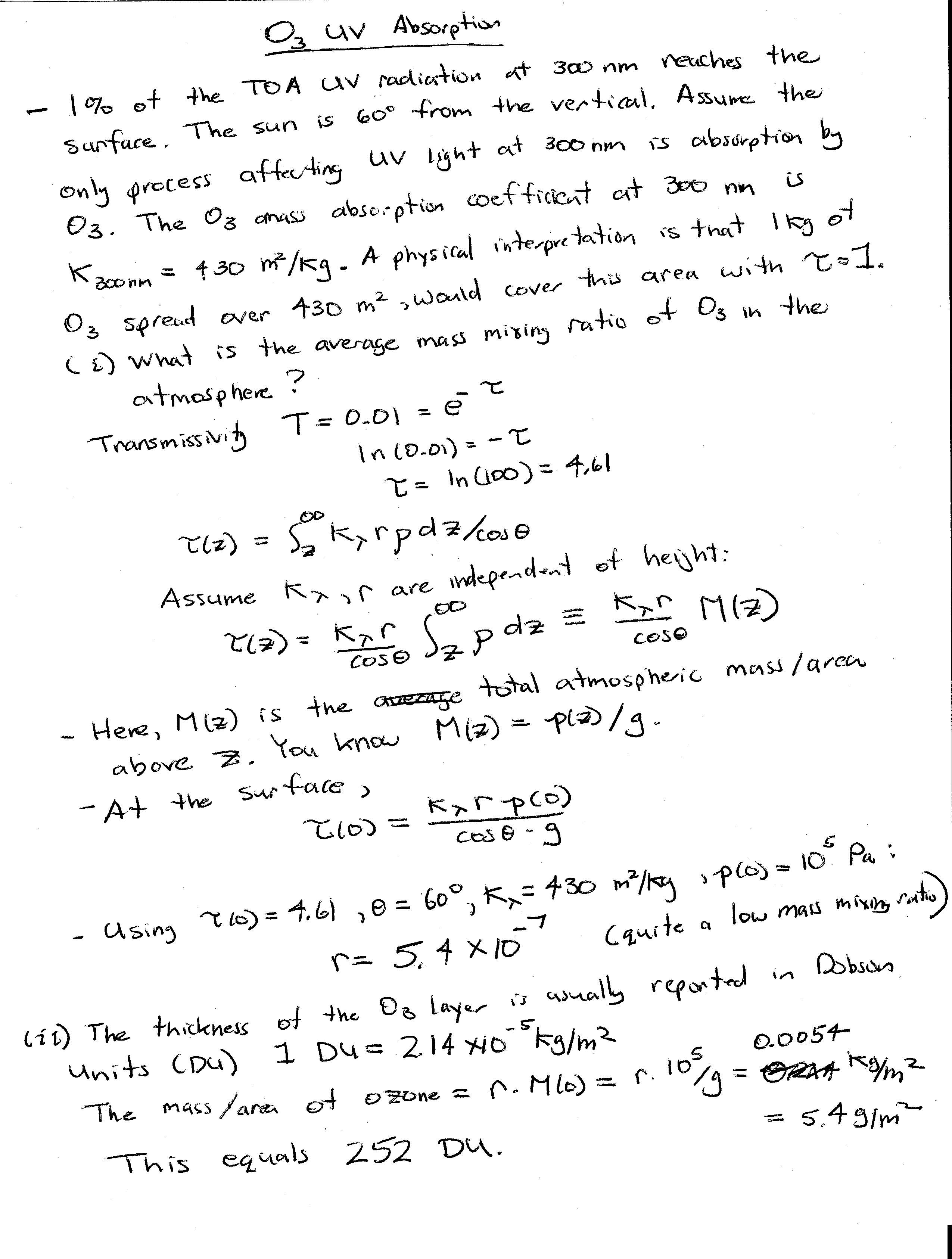# A more detailed look at what happens to solar energy as a function of latitude ...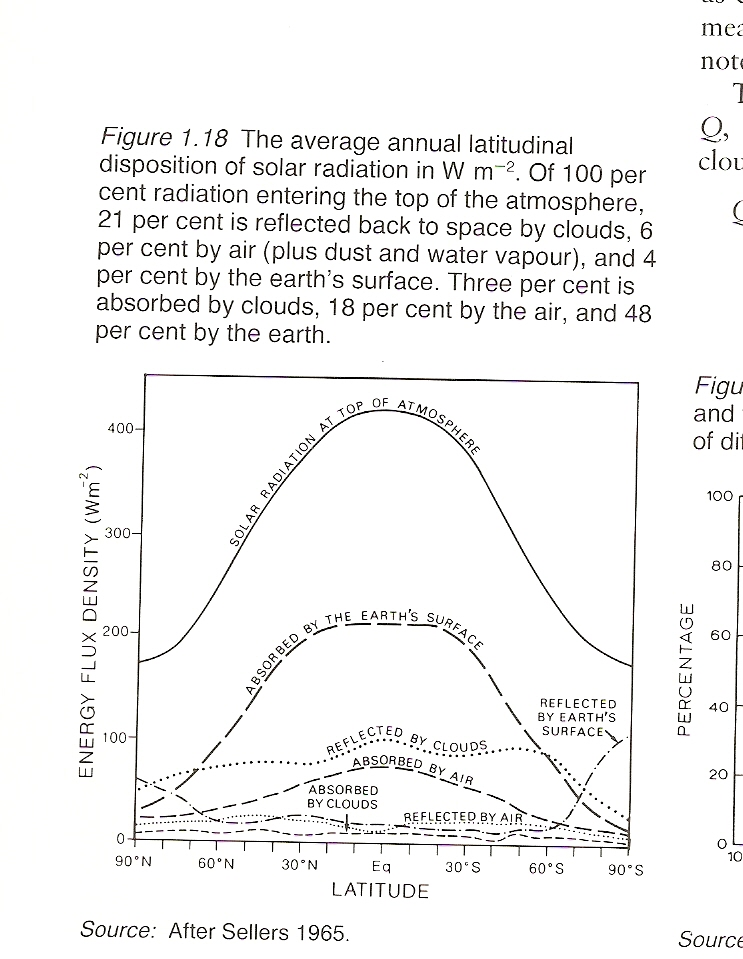# What Makes a Gas a Good Greenhouse Gas?

1) It must have absorption lines at frequencies where the earth emits thermal energy. One reason why CO2 is a good greenhouse gas for earth is because it absorbs thermal energy at the peak of the earth's Planck function.

2) Are there other absorbers already present? If so adding additional absorbers at that frequency won't make much difference.

3) How much of the absorber is already there? The radiative forcing per molecule is highest when you first add it, then decreases as you add more and more.

4) How many absorption lines does the molecule have? More complex molecules will have more absorption lines (more ways to vibrate or rotate) so will be better greenhouse gases. CFC's are perhaps the prime example. On a per molecule basis their radiative forcing of CFC-11 is about 10,000 times that of CO2. It turned out that banning CFC's in the interest of ozone depletion has probably played some role in slowing down climate change in the past 2 decades.

5) The height the molecule being added. Generally, the higher the temperature contrast between the surface and the molecule (i.e. the ambient temperature at the emission height), the better a greenhouse gas the molecule will be.

# Earth Energy Budget Plot

Notice how the 9.6 micron O3 band and the 15 micron CO2 band give rise to increase absorption at these wavelengths. Notice also that wavenumbers lower than 2000 cm-1 are in the LW part of the spectrum, so the plot above is entirely LW. Note that rapid increase in absorption as you go lower than 0.32 microns. This is due to the absorption of UV light by ozone.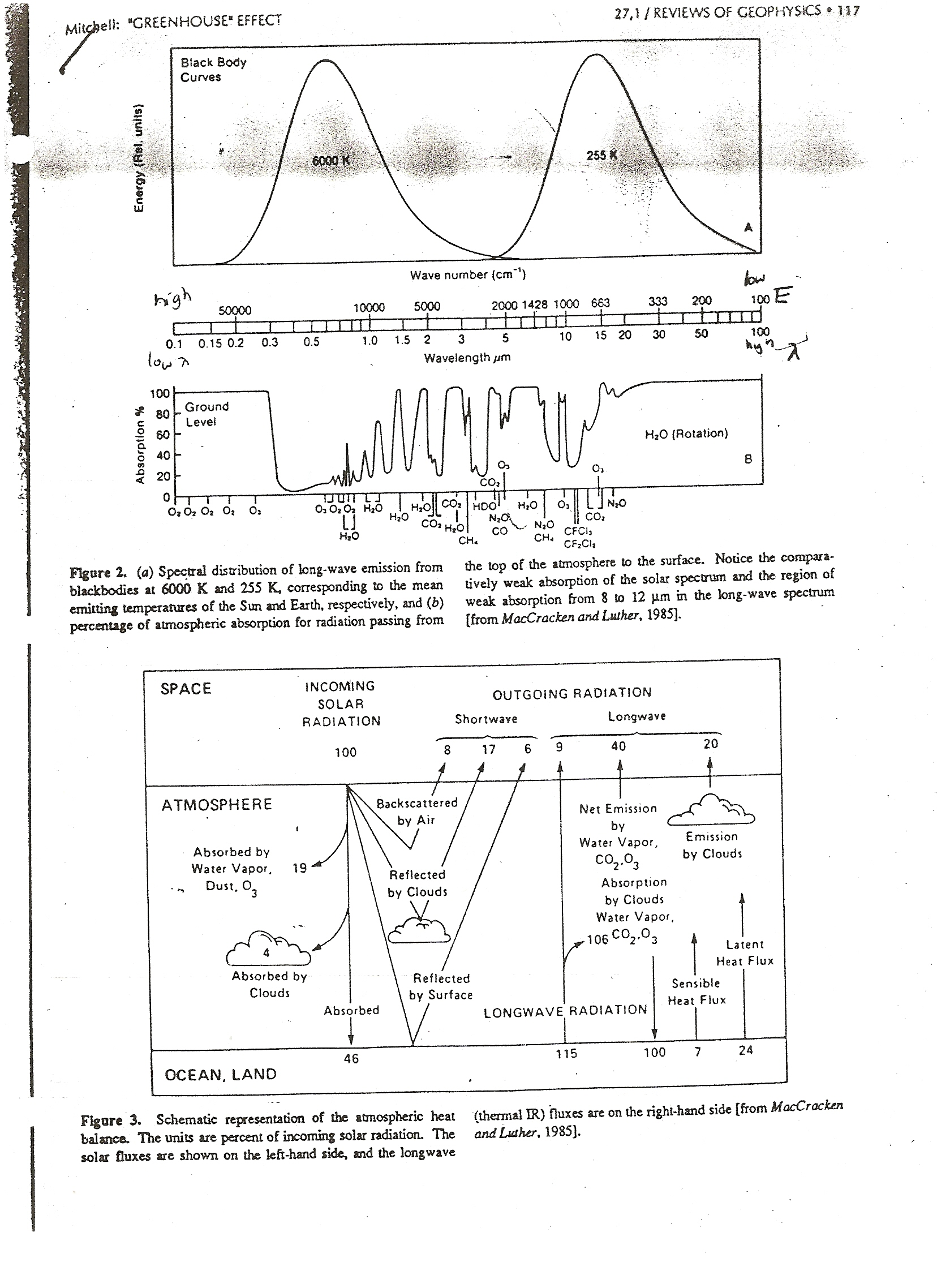You should know how to use this lower plot to estimate the solar reflectivity of the earth, the solar absorptivity of the atmosphere, and the long wave emissivity of the atmosphere. Note that 100 incoming solar units equals a global average of 340 W/m2, so to convert from the units here to W/m2, multiply by a factor of 3.4.

# Earth Energy Budget Plot - in W/m2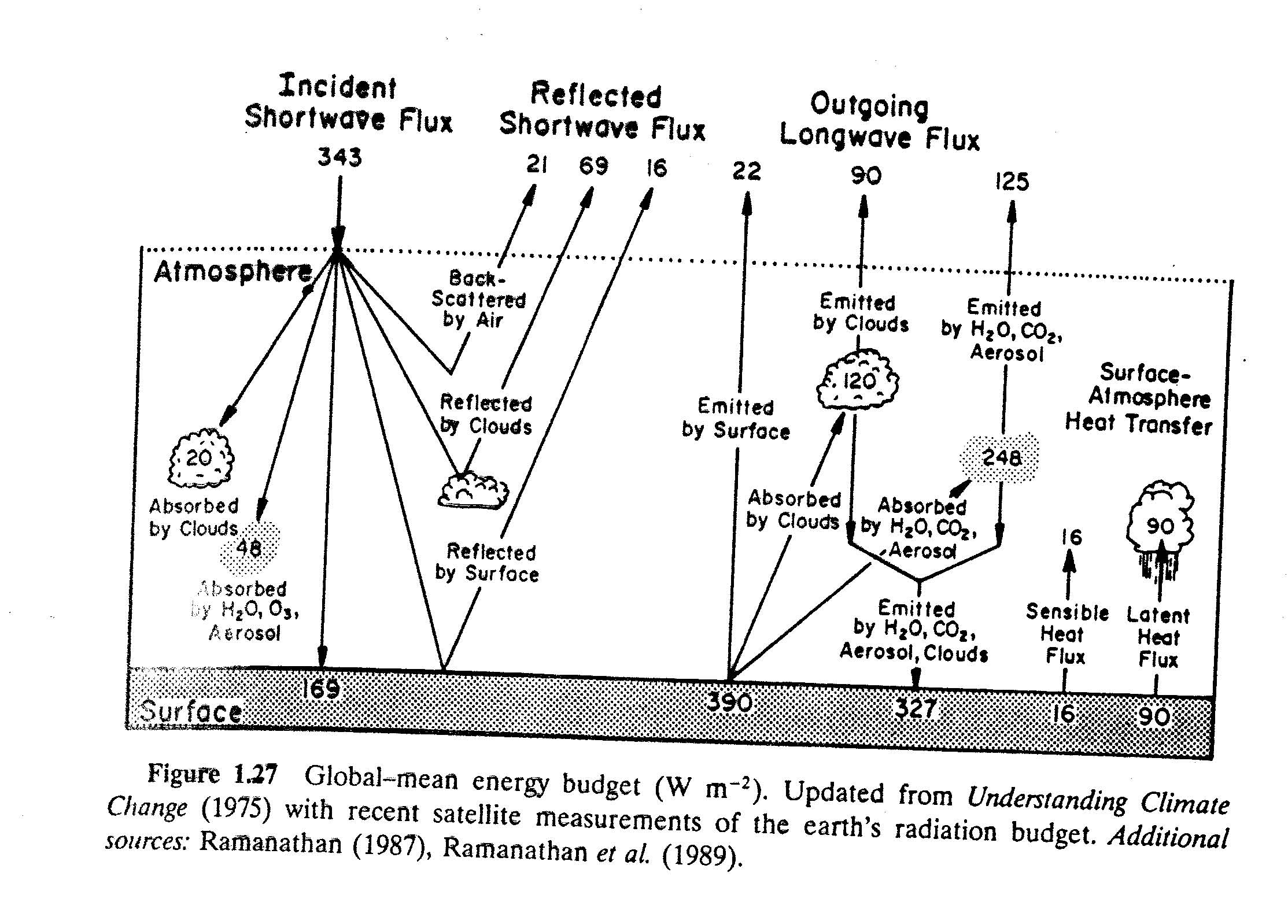In steady state, there must be a balance in 3 distinct energy budgets.

(1) TOA Energy Balance:

Energy into the Planet from Space = Energy out from the Planet to Space.

SWin + LWin = SWout + LWout.

343 + 0 = (21 + 69 + 16) + (22 + 90 + 125)

343 + 0 = 106 + 237

(2) Atmospheric Energy Balance:

We also have to take into account the surface heat fluxes of heat to the atmosphere:

SWin + LWin + Sensible Heat Flux + Latent heat Flux = SWout + LWout

(20 + 48) + (120 + 248) + 16 + 90 = 0 + (90 + 125 + 327)

542 = 542

(3) Surface Energy Balance: SWin + LWin = LWout + Sensible Heat Flux + Latent heat Flux

169 + 327 = 390 + 16 + 90

496 = 496

# SW versus LW

This difference is fundamental: SW photons come from the sun while LW photons come from an object on the earth. The average temperatures of the sun (6000 K) and earth (255 K) are so dissimilar, that their black body spectra have no overlap. If a photon has a wavelength larger than 4 microns, it is LW. If it's wavelength is shorter than 4 microns, it is SW.

It makes sense to talk about absorption and scattering of SW photons in the atmosphere. It does not make any sense to talk about emission of SW photons in the atmosphere: the black body emission spectrum of any object in the atmosphere is essentially zero at wavelengths less than 4 microns.

It makes sense to talk about emission and absorption of LW photons in the earth's atmosphere. We do not not usually talk about scattering of LW photons in the earth's atmosphere. Why? Mainly because the size parameter x is usually too small. A typical LW wavelength is perhaps 15 microns (near the peak of the earth's LW spectrum). Scattering could occur either via molecules (r ~ 1 nm, x ~ 0.0004), aerosols (r ~ 100 nm, x ~ 0.04), cloud droplets (r ~ 10 microns, x ~ 4), cloud ice crystals (r ~ 100 microns, x ~ 40), or raindrops (r ~ 1000 microns, x ~ 400). (See Figure 4.11 of the text for more details). LW scattering by molecules and aerosols does occur, but the small value of x makes the scattering efficiency sufficiently small that this process is not relevant to climate. LW scattering by clouds and ice crystals is of borderline relevance. Emission and absorption of LW energy in clouds is much more important. LW scattering by raindrops would be important when it is raining, but again, raindrops would be efficient absorbers of LW photons.

# Guam Spectral LW Emission Image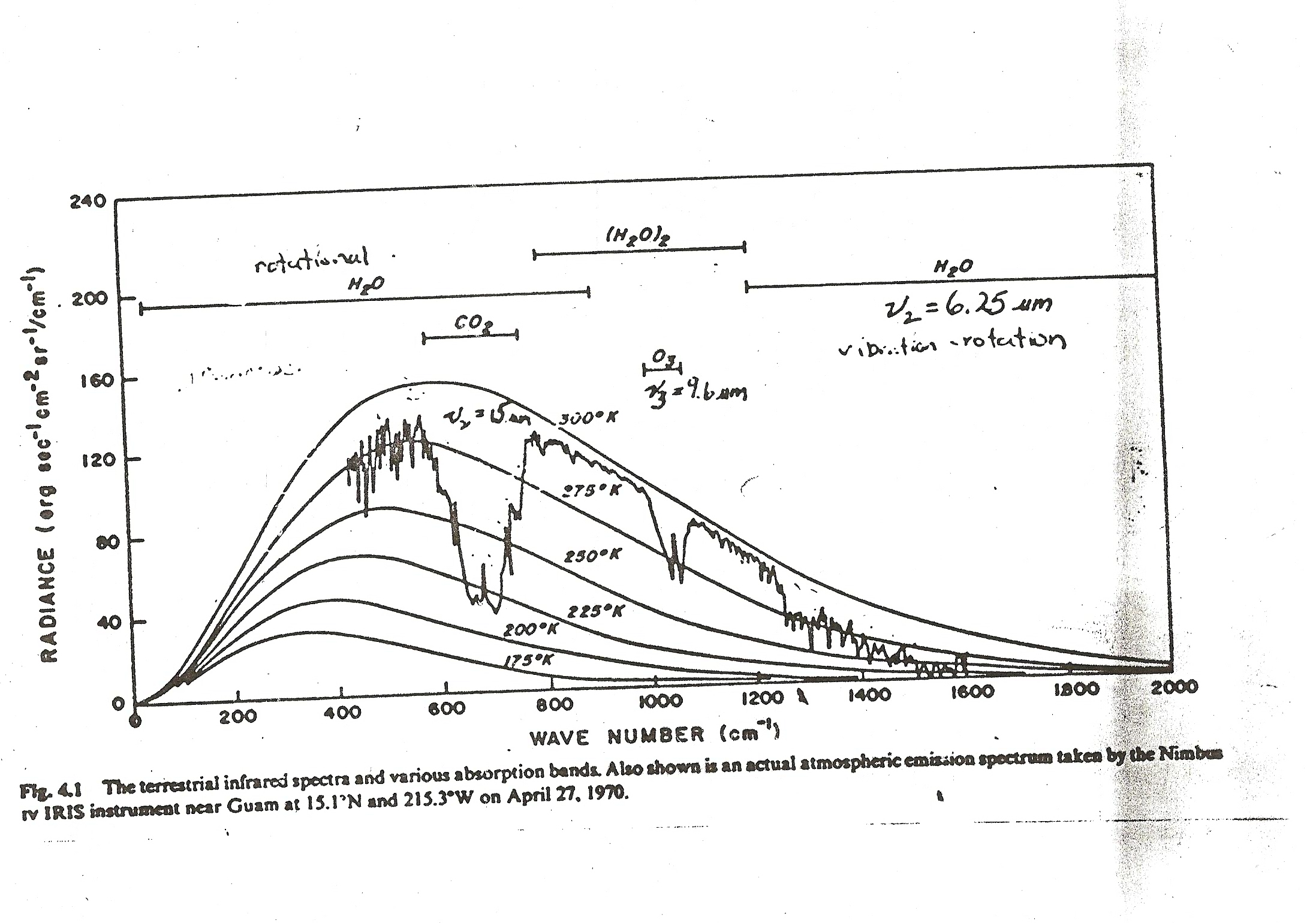Notice that the horizontal scale is wavenumbers or cm-1. Small wavenumber corresponds to low energy, while large wavenumber corresponds to higher energy.

This plot shows the LW radiance a satellite would measure from space looking down at the surface, when no clouds are present, over Guam. In the absence of greenhouse gases in the atmosphere, the satellite would observe a black body curve close to 300 K (likely the approximate surface temperature). If you look at the so-called window region between 800 cm-1 and 1200 cm-1, this is approximately what you see. In this region, there are very few greenhouse gases that emit and absorb LW radiation - with the exception of ozone near 1050 cm-1 (or 9.6 microns).

Near their absorption bands, greenhouse gases absorb the warm radiation upwelling from the surface, and replace it by colder radiation of reduced intensity, corresponding to the local temperature at that height. The dominant absorption feature in this image is the 15 micron CO2 band. Most of this emission is coming from CO2 molecules in the upper troposphere and lower stratosphere. In the tropics, the average temperature of the tropical tropopause is about 195 K, so it is hard for the CO2 emission to go below the 200 K black body curve, even if most of the emission to space at a particular wavelength (dF_lambda/dz) is emitted from a height region close to the tropopause.

There is a narrow spike in CO2 at the center of the CO2 band. This occurs because, at this wavelength, where the absorption cross-section of CO2 is largest, most of the emission to space is coming from the stratosphere, where the temperature increases with altitude, and it is warmer than temperatures near the tropical tropopause.

The rotational and vibrational bands of water vapor are also clearly very important over a broad range of wavelengths.

Measuring the black body emission spectrum of a planet or star is a good way of getting insight into the composition of its atmosphere.

# LW Emission Spectra from Different Regions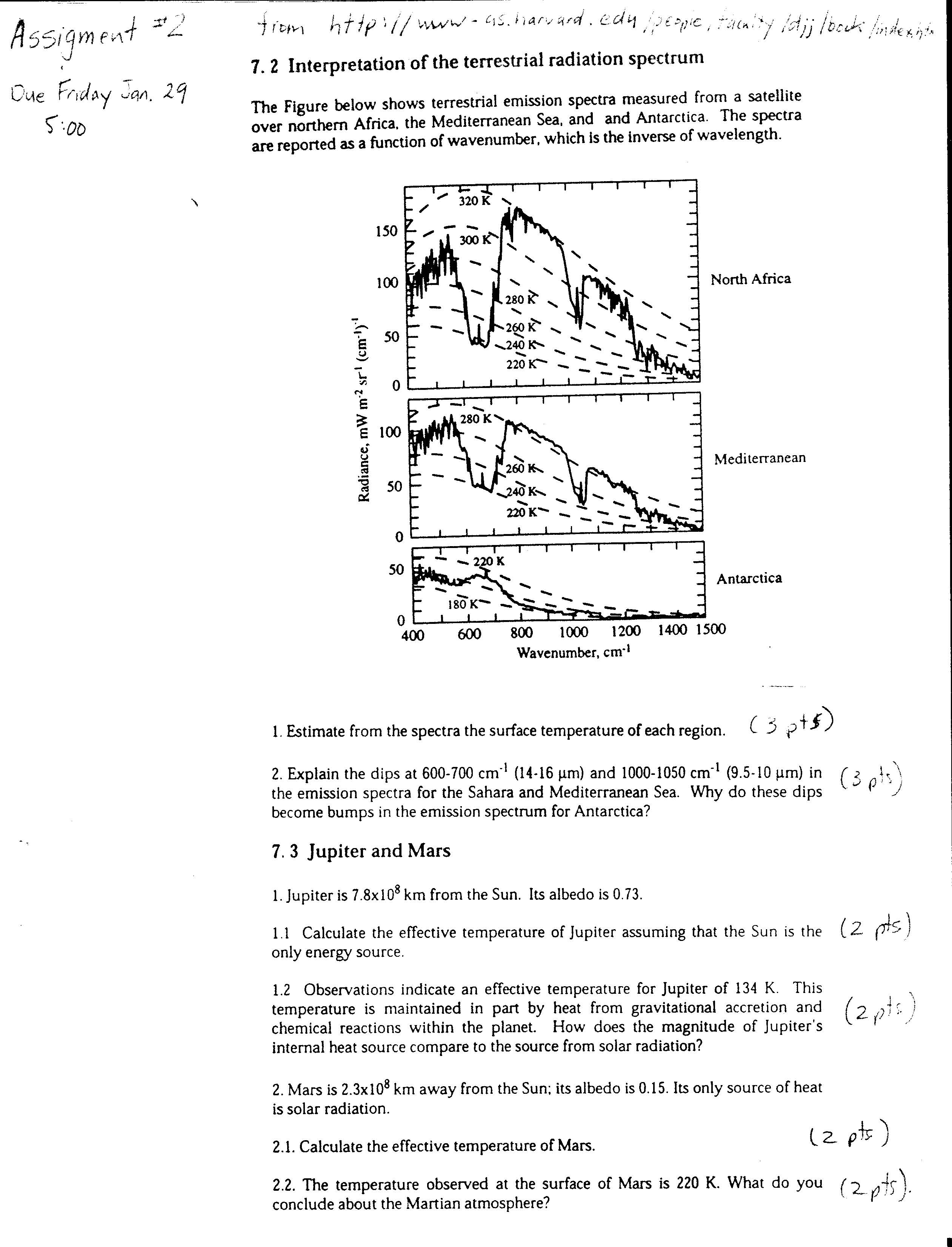The LW emission spectra can vary dramatically depending on the surface temperature and whether clouds are present. This figure shows clear sky LW spectra from three regions. The surface of Antarctica appears close to 180 K (!). In this case, since the surface is so cold, CO2 is increasing the LW emission to space.

Given the value of the solar flux S0 of a planet, and its solar reflectivity, one can calculate its TE. An average surface temperature Ts > TE does not always imply that there is a greenhouse effect. The planet could have an internal heat source. For example, a large planet could be undergoing gravitational compression - this decrease in gravitational potential energy would be converted to heat. Another possible heat source is internal radioactive decay. On earth this is very weak, but significant since it maintains mantle convection, and the movement of tectonic plates and volcanism.

# LW Emission Spectra from a Cold Cloud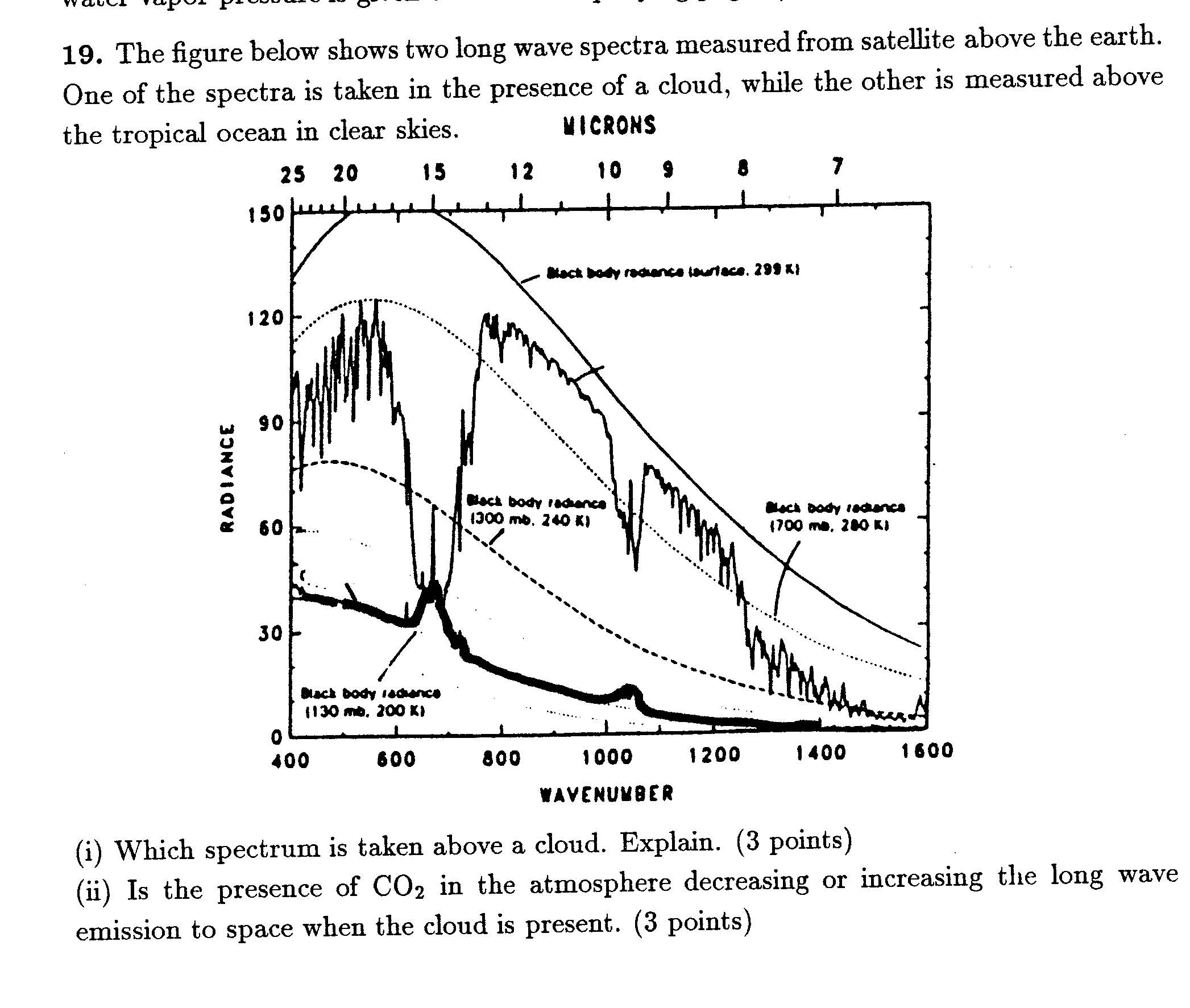The LW emission shown as the thick line was taken when a cloud was present. The cloud is acting like a grey body (or close to a black body). There seems to be no trace of the warm LW emission from the surface. The cloud top is clearly significantly colder than 240 K, since it lies significantly below the 240 K dashed black body curve. Clearly, high cold clouds can dramatically reduce the LW emission to space, and be powerful greenhouse agents (acting like emergency blankets).

The peaks in the cloud spectra refer to LW emission from CO2 and ozone in the stratosphere. This emission is coming from a height range in the stratosphere which is warmer than the cloud top, so that CO2 and O3 are increasing the LW emission to space (functioning as anti-greenhouse gases in this instance).

# Absorption Lines

Some of the thermal radiation emitted by the surface goes directly to space, especially in the so-called "window" region. (But note that the atmosphere is only transparent to LW radiation in this wavelength region when no clouds are present.) Radiation at other frequencies (or wavelengths) is much more likely to be absorbed. Greenhouse gases absorb only at special frequencies (or wavelengths), corresponding to resonant energies at which they vibrate/rotate. These are called absorption lines. A satellite looking at the thermal radiation emitted by the earth sees decreases in radiance at these absorption lines. This is because the "warm" thermal radiation emitted from the surface is absorbed at these wavelengths, and is replaced by "colder radiation" (reduced radiance) coming mainly from gases in the atmosphere at a temperature colder than the surface. This reflects the insulating behavior of greenhouse gases.

In order for greenhouse gases to increase the surface temperature, the atmosphere must be colder than the surface. Otherwise greenhouse gases would increase the emission of thermal energy to space. For example, there can be no greenhouse effect on a planet with an isothermal atmosphere.

Greenhouse gases emit and absorb LW radiation only at very specific frequencies or lines. However, in the liquid or ice form, as in a cloud, water becomes a grey body and has an emissivity which is only weakly wavelength dependent. This is a deeply strange result: why would a water molecule behave so differently depending on whether it is in the gas phase or a liquid? Perhaps the easiest way of explaining this is that the energy levels of water molecules are smeared out by the closer presence of the other water molecules in the liquid or ice phase, so they are no longer independent oscillators (springs), but can interact with the radiative field at a continuum of energy levels.

# What is cloud Liquid Water Path (LWP)?

Cloud LWP is the depth of the puddle of water you would make by collecting all the cloud droplets together. Most clouds do not contain very much water. In fact non-precipitating clouds typically have an LWP of 0.1 mm. This is remarkably small, and demonstrates that dividing liquid water into tiny droplets can greatly magnify the scattering of visible light by the cloud. Precipitating clouds can have much larger LWP's.

# Mysterious Factor of 4 in S0/4

The area of the sun's radiation that the earth intersects is pi times the radius of the earth squared. The total area of the earth is 4 times pi times R squared. The global 24 hour average TOA (Top Of the Atmosphere) solar flux is therefore S0/4. In constructing a simple one layer climate model, all fluxes have to be global averages. Therefore, S0/4 is the appropriate TOA solar flux.

However one does not always automatically divide S0 by 4 in every case. In the case of a directly overhead sun, the TOA solar flux is S0. In the case of solar zenith angle of theta, the TOA solar flux is S0 times cos(theta). Try and determine from the question whether is is based on a particular solar zenith angle theta, or is a climate type problem where it is more appropriate to use S0/4 (i.e. globally averaged as opposed to instantaneous values of solar flux).

# One Layer Grey Atmosphere Toy Model

If a planet did not have an atmosphere, and no internal heat source, its average surface temperature would equal its TE ( = 255 K for the earth). The purpose of this model is to show that an atmosphere with greenhouse gases will give rise to a surface temperature Ts > TE, and a atmospheric temperature Ta < TE.

The main defect of the One Layer model is that it over predicts the surface temperature, since it does not consider solar absorption in the atmosphere.

The Global Energy Budget Figure shows that 106 of the upwelling 115 units of LW radiation from the surface is absorbed in the atmosphere. This gives a LW absorptivity due to greenhouse gases and clouds of 0.92. The one layer model (with no solar absorption in the atmosphere) predicts that the mean surface temperature of earth in this case should be 297 K. The actual global mean temperature is 288 K, so this model overestimates the surface temperature by 9 K.

The main reason for the overestimate is that the One Layer model assumes that all solar energy not reflected to space is absorbed at the surface. Figure 3 shows that 46 units of solar energy are absorbed at the surface and 23 in the atmosphere. Solar absorption in the atmosphere increases the temperature of the atmosphere, and therefore decreases the effectiveness of greenhouse gases.

Solar absorption in the atmosphere also decreases rainfall, by making the atmosphere more stable - it is harder for clouds to rise up through a warmer atmosphere.

# Spectral response to Doubling CO2

FIXED RESPONSE:

If you double the concentration of CO2 in the atmosphere, but keep everything else about the atmosphere fixed (clouds, water vapor concentrations, the surface temperature), you will reduce the outgoing LW flux to space by about 4 W/m2. The top plot gives an idea of the wavelengths (or wavenumbers) at which this reduction in LW flux is coming from. It represents the change in the LW emission to space from a CO2 doubling as a function of wavelength. The center of the CO2 band is 750 cm-1. Doubling CO2 in a fixed atmosphere decreases CO2 emission at most wavelengths in the band, as you would expect, but increases CO2 emission at the center of the band. Why?

At any wavelength, doubling CO2 increases the effective height of average emission to space at that wavelength, i.e. moves the peak of the weighting function to a higher altitude. At the center of the band, where the absorption coefficient of CO2 is largest, the height of maximum emission to space is occurring at the highest altitude. This wavelength is labeled "1" to be consistent with the notation of Figure 4.33. At this wavelength, where the absorption cross-section of CO2 is largest, the CO2 emission to space is not coming from the troposphere, but from the stratosphere where temperatures increase with altitude. Figure 4.33 shows that this weighting functions peaks near 30 hpa, or 25 km. The emission to space at the center of the CO2 band will increase when CO2 is doubled because this emission will now come from an even warmer temperature (i.e. at an even higher stratospheric altitude). At the sides of the band, where the emission is coming from the troposphere, increasing the average height of emission reduces the average emission temperature and reduces the intensity at these wavelengths.

If you were to average over all wavelengths, you would get an overall reduction in LW radiation to space (~ 4 W/m2) from doubling CO2 in a fixed atmosphere. This is an integral over the center of the band where emission is increased, and at the sides, where emission is reduced. This reduction in LW emission causes an energy imbalance in the earth: total emitted LW to space from the earth is no longer equal to the total absorbed SW by the earth. The lower figure shows how the atmosphere responds to this imbalance.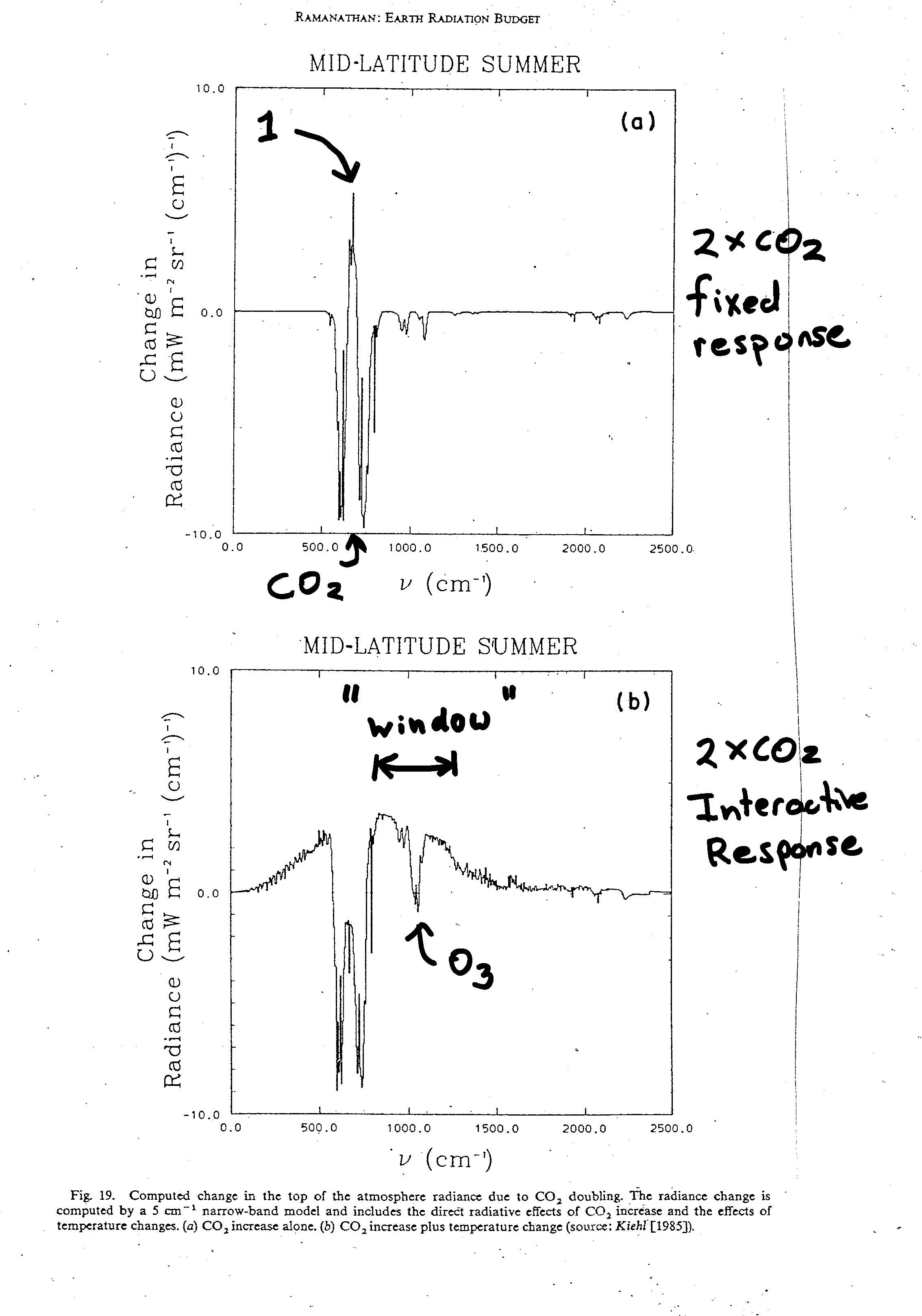INTERACTIVE RESPONSE (Could also call Steady State Response):

The climate system must respond to any energy imbalance. The lower plot shows how the LW emission to space changes in response to the atmospheric changes triggered by an energy imbalance from a CO2 doubling. Surface and atmospheric temperatures and water vapor concentrations have been allowed to change in such a way to reach a new energy balance. The stratosphere clearly cools: The LW emission at the center of the CO2 band goes down. This is driven by an increase in LW cooling in the stratosphere due to the increased CO2 (see explanation below). Unless this increased LW cooling were somehow compensated by an increase SW ozone absorption, stratospheric cooling must occur, and it does (both in this model and the real atmosphere).

There is increased emission to space in the window region between 750 and 1500 microns (except for the ozone band in the middle). This is the region where, in the absence of clouds, the atmosphere is quite transparent to LW radiation, so that the LW intensity to space is a direct indication of surface temperatures. By increasing the surface temperature, and increasing the LW emission to space in the window regions, the earth is trying to compensate for the reduced LW emission to space within the CO2 band. Provided there is no change in solar absorption, the change in LW radiance integrated over all wavelengths must be zero to maintain energy balance: if you integrate the change in radiance over all wavelengths in the lower plot, you should get zero. The window region functions as the earth's radiator fins. They are the way the earth can directly get rid of excess energy by warming the earth's surface (Of course these radiator fins are prevented from working when there are clouds.)

This figure helps demonstrates the importance of stratospheric cooling to the greenhouse effect: if the stratosphere did not cool, so that doubling CO2 increased LW emission to space from the stratosphere, you would not need as much surface warming to bring about a new energy balance.

# Why are increased CO2 concentrations expected to continue to cool the stratosphere?

Stratospheric temperatures have been decreasing for several decades. This is consistent with an increased greenhouse effect. Why?

From the energy budget figure above, the atmosphere absorbs 106 units of LW radiation but emits 160. This 54 of net LW atmospheric cooling is balanced by 7 units of sensible heat flux, 24 units of latent heat flux (condensational heating), and 23 units of solar absorption. Solar absorption is therefore a significant term in the energy budget of the atmosphere, especially in the stratosphere where condensational heating is zero, and there is a direct balance between solar absorption and net LW emission. The main solar absorber in the stratosphere is ozone. Water vapor concentrations in the stratosphere are extremely low, so that the main greenhouse gas is CO2. Increasing CO2 increases the rate of LW cooling. In the absence of any way of increasing the SW heating rate, the only way for the atmosphere to maintain energy balance is to reduce temperatures in the stratosphere.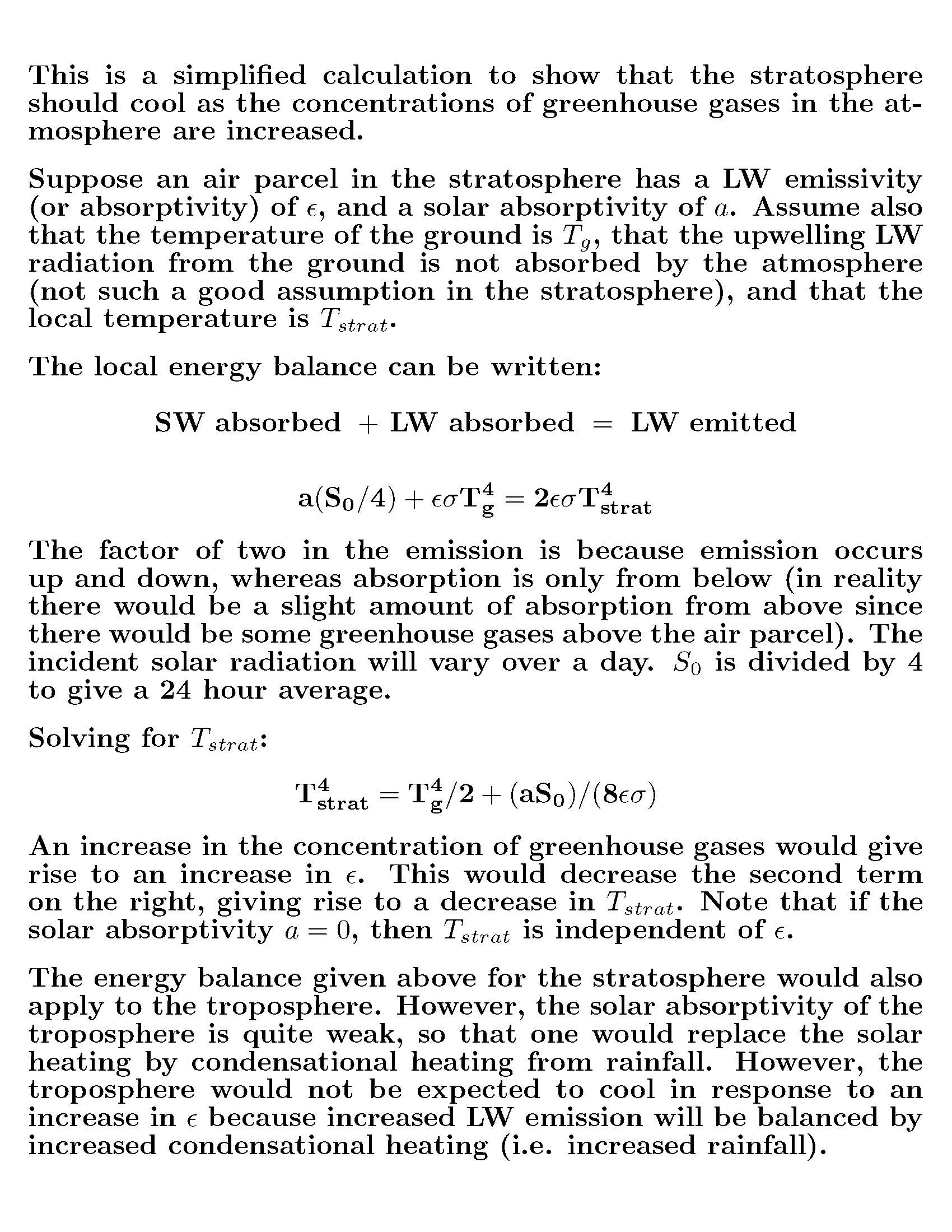In the above calculation, the solar absorption "a" in the stratosphere is due to ozone. The observed stratospheric cooling is therefore likely due to some combination of ozone depletion and CO2 increases, but the dominant reason for the temperature decrease would be the CO2 increase.

It might strike you as inconsistent that increasing the concentration of a greenhouse gas tends to cool the atmosphere (increasing LW emission) but warms the surface. But actually, this is quite consistent. If you wear a winter jacket, you want it to be well insulated so that your body heat does not easily diffuse to the outside surface of the jacket. By keeping the outside surface of the jacket cold, you reduce LW emission to your surroundings, and stay warm.

# Absorption coefficient = Emission coefficient

This only applies to greenhouse gases and clouds in the LW part of the spectrum. For example, the LW emissivity of a cloud will equal the LW absorptivity of a cloud, but will not equal the SW absorptivity of the cloud. Also note that Kirchoff's law does not imply that the LW flux emitted by a cloud equals the LW flux absorbed by a cloud. It refers to the coefficients (emissivities) only.

# The Enhanced Greenhouse Effect (2 X CO2):

Suppose that the concentration of CO2 in the atmosphere were to double. On average, one would expect the longwave emission that reaches space from emission from CO2 molecules in the atmosphere to originate, on average, from a higher altitude. In general, temperature decreases with altitude. Increasing CO2 therefore tends to reduce the emission of longwave radiation to space (all other factors being equal). This "no feedback" reduction in outgoing thermal radiation to space has been calculated to be 4 W/m2 (using complex radiative transfer models of atmospheric emission, absorption, and scattering). In the absence of any change in the solar budget (i.e. no change in the solar reflectivity of clouds), thermal and solar budgets would be out of balance. There would be more SW energy coming in than LW energy going out. The only way to bring the LW and SW budgets back into balance is to increase the surface temperature. This also increases the temperature of the atmosphere (since the lapse rate of the atmosphere remains approximately moist adiabatic, at least in the tropics), so that LW emission from the surface and atmosphere both go up.

A radiative flux of 4 W/m2 is the amount of heat you feel standing about a 1.1 meters from a 60 W light bulb. What would happen if you REMOVED all CO2 from the atmosphere? Would you INCREASE thermal emission to space by 4 W/m2? No. It turns out that the radiative forcing of CO2 is highly nonlinear. Removing all CO2 from the atmosphere would actually increase outgoing thermal by around 25 W/m2!! This would cause a huge catastrophic surface cooling, as the surface temperature reduced in an attempt to bring the global energy budget back into balance (likely a snowball earth). And this calculation does not even take feedbacks into consideration. In general, the radiative forcing of a greenhouse gas depends non-linearly on its atmospheric concentration. When the gas is already present in high concentrations, adding more makes less difference. The absorption lines become saturated, and CO2 has a smaller climate impact. This is shown by the first two figures below in the Climate Dynamics Chapter.

Why do atmospheric scientists often prefer to associate the effect of a greenhouse gas concentrations with a change in radiative forcing, as opposed to a change in surface temperature? (i.e. doubling CO2 reduces LW emission to space by 4 W/m2). The radiative forcing of a greenhouse gas is the effect of a gas on the outgoing thermal radiation calculated by keeping atmosphere fixed at its current state. This "no feedbacks" calculation is quite unambiguous and straightforward. Scientists can agree on it, since we can agree how LW radiation propagates through the atmosphere. The actual effect of some change in greenhouse gas concentrations on surface temperature, after you have included feedbacks, is complex, and model-dependent, mainly because climate scientists have so many problems dealing with clouds. Hopefully some of these model uncertainties will diminish over time.

One way of viewing the greenhouse effect: provided the energy from the sun doesn't change, and the solar reflectivity of the earth doesn't change, the net thermal emission to space by the earth (combined surface and atmosphere) can't change. This implies that the net area under the curve shown in Figure 2.4 (Guam) can't change (treating it as if it were representative of a global average). If the level of CO2 in the atmosphere is increased, outgoing radiance within the 15 micron absorption line is reduced. To keep the net LW emission to space constant, this means that the outgoing radiance at other wavelengths must increase. It can most easily do this at wavelengths in the window region where the thermal radiation goes directly to space without being absorbed by the atmosphere. Since radiation in this window directly reflects surface temperature, an increase here implies the surface temperature is also increasing.

# Spectral Cooling Plot (not responsible for)

This figure shows the importance of CO2 and O3 LW cooling in the stratosphere, and also that water vapor dominates in the troposphere (below 200 hPa). The 15 micron (800 cm-1) CO2 band dominates in the stratosphere. The 9.6 micron (1000 cm-1) ozone band also plays a role. Keep in mind 1 mb = 1 hPa, and 100 hPa is close to 16 km, 10 hpa close to 32 km, and 1 hPa close to 50km. Notice that there are altitude regions where CO2 and O3 actually heat the atmosphere locally (the grey regions where there is a negative cooling). These are height ranges where the local temperature is very cold, so that LW emission is reduced. Net absorption of LW radiation from below is larger than upward and downward emission, so there is a net warming.

This plot should be compared with Figure 4.29 in the text. Note for example, from Figure 4.29 that ozone gives rise to a LW heating between 150 hPa and 10 hPa. This is roughly consistent with the pressure range shown in grey for ozone in the plot below (grey in the image below corresponds to a negative cooling, i.e. heating.)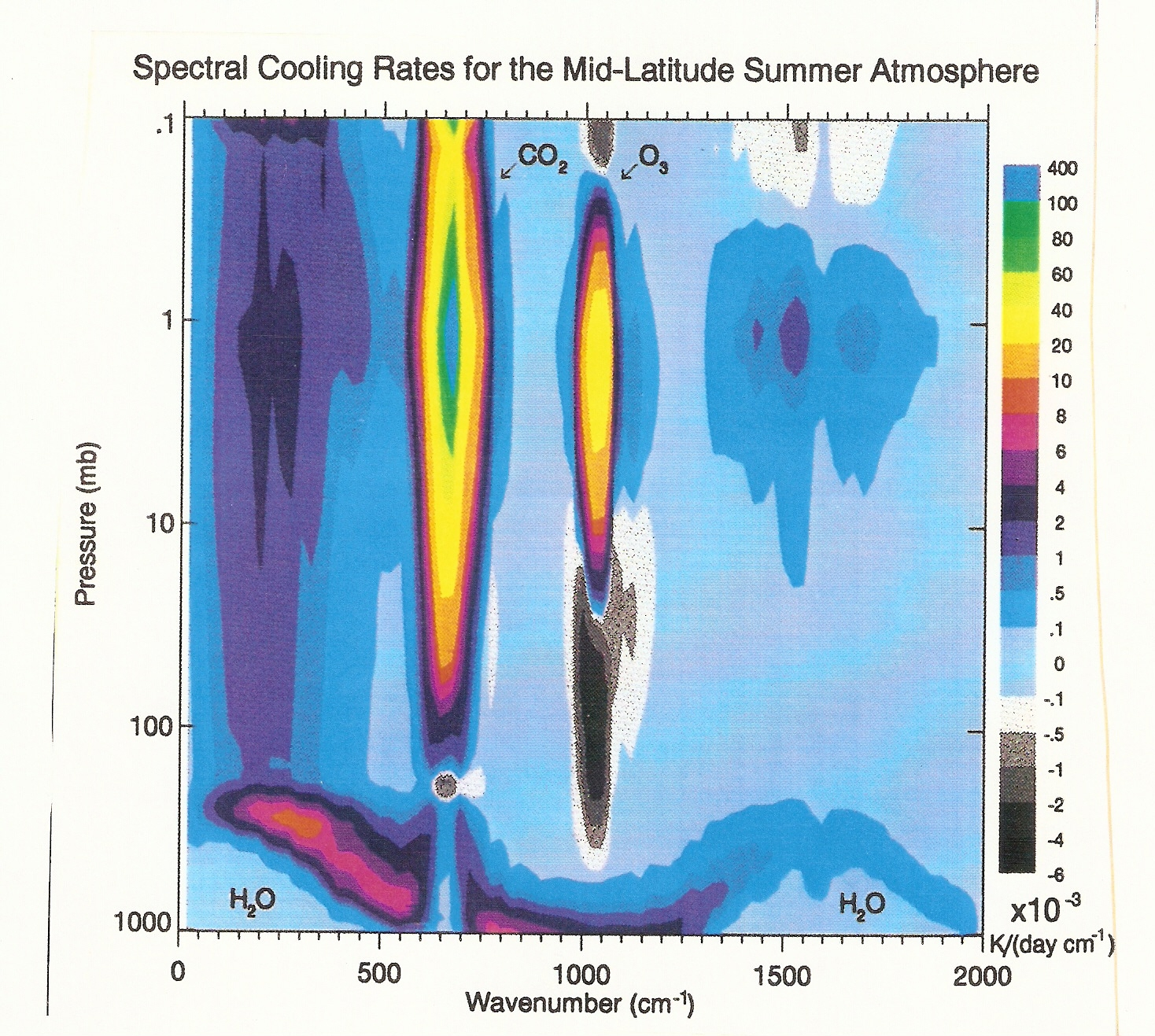# Weighting Functions

This discussion is intended to provide some context to Figure 4.33.

Suppose a satellite is measuring the upwelling LW radiance from the earth at a particular wavelength. If this wavelength is within an absorption band of a greenhouse gas, then the photons reaching the satellite will be coming from the atmosphere, but possibly also from the surface as well, depending on the strength of the absorption line, and the concentration of the greenhouse gas. This is expressed in Eq. (4.48).

The first term on the left is Bi(Ts)*exp(-tau). Bi(Ts) is the black body emission from the earth's surface, while exp(-tau) is the probability that a photon emitted from the surface will reach space, or the transmissivity at that wavelength.

The second term on the right of (4.58) represents atmospheric emission reaching space, integrated over all altitudes. It is the product of a weighting function w(z) and the blackbody emission at that height B[T(z)]. The weighting function gives an idea of the relative importance of a height range to the intensity as measured by a satellite. By comparing the two lower plots of Fig 4.33, you can see that the weighting functions go to zero as the transmissivity exp(-tau) at that wavelength goes to zero. For example, the photons in band 1 measured by the satellite must all have originated above 200 hPa. Weighting function 1 peaks near 20 hPa (~ 25 km). Weighting function 2 peaks near 50 hpa (~ 20 km). Both peaks lie in the stratosphere, where the temperature increases with altitude. The intensity at wavelength 1 as measured by the satellite must be higher than the intensity measured by the satellite at wavelength 2, since it is coming from a height range where the atmosphere is warmer.

Transmission Probability: In the atmosphere, every CO2 molecule is continuously absorbing ambient LW radiation at wavelengths near its absorption lines. It is also emitting LW radiation at its absorption lines, at a rate depending on its local temperature. Photons emitted by CO2 molecules higher up in the atmosphere have a greater probability of reaching space than those emitted lower down. The transmission probability T(z) - the fraction of the photons emitted by CO2 which reach space from a given height z - will reach 1 at the top of the atmosphere, and progressively decrease as you approach the surface. The plot on the bottom right of Figure 4.33 shows how the transmissivity T(z) depends on wavelength as the the absorption coefficient of CO2 is increased from 1 - 6.

The mixing ratio of CO2 is roughly independent of height in the atmosphere (now about 380 ppmv). The total molecular density of air decreases exponentially with height - air gets thinner as you go higher up. This means that the CO2 density decreases approximately exponentially with altitude (even though the mixing ratio is constant). This is why all the weighting functions got to zero at high altitude.

# Increasing the CO2 Concentration moves the peaks of the Weighting Functions to a higher altitude.

Weighting functions go to zero at high altitude because the concentration of the greenhouse gas gets very small as the atmosphere becomes very thin. Increasing the concentration of a greenhouse gas therefore increases the value of its weighting function at high altitudes. Weighting functions tend to go zero as you approach the surface because the transmissivity is going to zero. Increasing the concentration of a greenhouse gas decreases the transmissivity even more, therefore reducing the weighting functions at low altitude. The combination of the high altitude increase + low altitude decrease shifts the weighting function to a higher altitude.

# Trenberth Energy Budget Paper

Important parts to know:

Figure 1: Where does 0.9 W/m2 come from? A Radiative Forcing is a change in SW(in) - LW(out). Greenhouse gases increase the emissivity of the atmosphere, decrease LW(out), and have a positive radiative forcing. (Top four rows). The next four rows represent possible changes in SW(in). For example an increase in aerosols from pollution would scatter more solar radiation back to space, decrease SW(in), and be a negative radiative forcing. Satellite measurements suggest that any change in SW(in) from a change in S0 (or TSI) is small. The "Radiative Feedback" is what could be called the "Interactive Response" - respresenting the change in LW(out) due to increases in surface temperature and changes in water vapor. This is the change in LW(out) due to a climate response of the earth trying to reach a new balance in which SW(in) = LW(out). Also shown is an increase in SW(in) as estimated from changes in snow/ice cover - known as the ice-albedo feedback. The total net imbalance is likely about 0.9 W/m2. Note that this number is not obtained from actual global average top of the atmosphere measurements of SW(in) and LW(out) over time, but is a sum over many terms that might affect this balance.

The earth can do many things with this surplus 0.9 W/m2. These are discussed in the first paragraph of the paper (you should be familiar with these). The surplus need not neccessarilly be used to increase the mean surface temperature. So the absence of an increase in surface temperature since 1999 is not inconsistent with the surplus heat, but we would like to know where it went. The paper is an assessment of our current understanding of the various possibilities, But unfortunatley, due to a lack of the right observing network, we don't know how to close the earth's energy budget.

# Optical Depths in the Visible/UV

The vertical axis is optical depth from the Top of the Atmosphere (TOA) to the surface (sometimes called optical thickness). Note that the scale is logarithmic. If tau is less than 0.1, one considers the atmosphere to be basically transparent, if tau is between 0.1 and 2, the atmosphere is partially transparent, whereas if tau is larger than 2, the atmosphere is essentially opaque at that wavelength.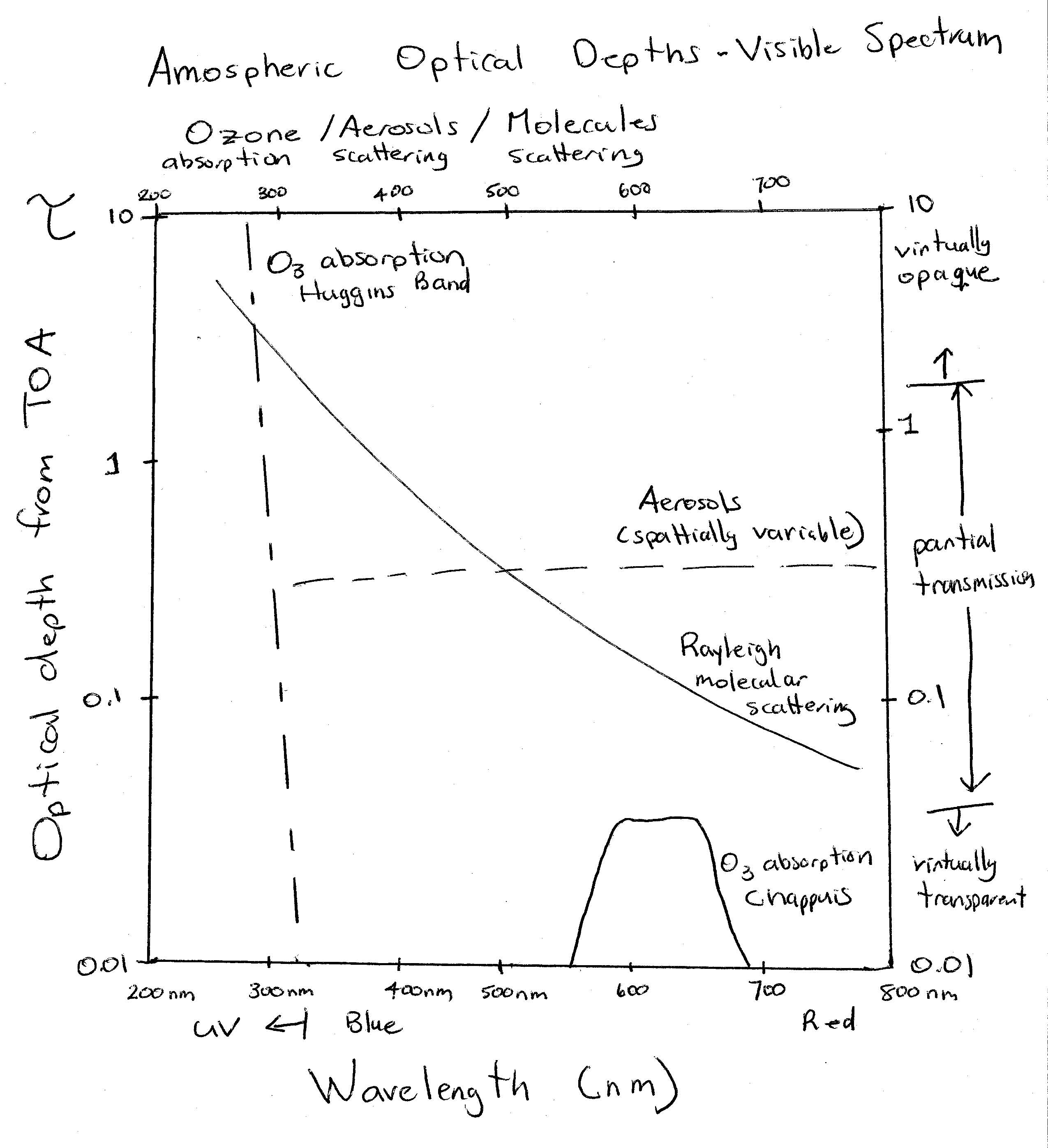Molecular: Rayleigh scattering has the classic 1 over lambda to the fourth power dependence. The optical depth of Rayleigh scattering would depend on solar zenith angle - i.e. smaller for overhead sun than a sun lower in the sky. This plot would assume a particular Solar Zenith Angle (SZA); not sure what it is. The visible range is 380 nm to 780 nm. Our eyes have evolved to detect radiation in the maximum in the solar output of the sun since that is where the "signal" will be largest. It would be harder to see at 200 nm since there are so few photons at that wavelength, even though each photon would have a higher energy. The increased scattering of molecules in atmosphere at the blue (high energy) end of the visible spectrum makes the sky blue.

Ozone: The ozone absorption cross-section increases rapidly below 330 nm, so that by about 310 nm, ozone absorption is more important than molecular scattering in protecting us from UV light. It turns out that ozone is also a weak absorber of red light - the so called Chappuis band. Because this is weaker than Rayleigh scattering, it does not have much impact on the colour of the sun. The sun is yellow rather than white (its color in outer space) mainly because blue light is preferentially removed by molecular scattering.

Aerosols: The dashed line represents the scattering optical depth of aerosols. Aerosols both scatter and absorb solar light, but mostly scatter, unless they are very dirty, e.g. contain lots of soot. Aerosol scattering is highly variable in space and time; much higher in polluted regions, along the coast where there can be lots of sea salt aerosols, or downwind of forest fires. Note that the aerosol scattering is only weakly wavelength dependent. Aerosol scattering will therefore usually make the sky look white. This will be true unless the aerosols are very monodisperse - i.e. all have almost the same size, in which they can have a particular color.

# Scattering efficiency (Not responsible for)

The fundamental definition is (4.16). It can be thought of as the probability of an incident photon being scattered, where incident refers to photons whose path intersects the areal cross-section of the aerosol (or molecule). The areal cross-section is just a way of expressing the physical size of the object, e.g. 4 times pi times the radius squared for spherical droplets. You would think that this probability can never be higher than 1, but Figure 4.13 shows that it can be larger than 4. (strike you as weird? Just remember that photons are waves and can be deflected by objects that they don't "hit" ...). Also note that the scattering efficiency scales as the size parameter x to the fourth power for small x. This "Rayleigh regime" is not shown in Figure 4.13. The mass absorption coefficient and the scattering efficiency refer to the same physical quantity, but express it in different units.

# Why are aerosols (usually) white? (Not responsible for)

Figure 4.13 shows that the scattering efficiency is almost always a function of the size parameter x. This means that almost every object has a color: it will scatter some wavelengths more efficiently than others. The exceptions would be scattering objects with very large x (> 100), where the scattering efficiency converges to 2, or objects which are highly absorbing (mi > 0), like soot. In class, I showed that the size parameter x of cloud droplets in the visible spectrum (350 - 700 nm) is large: this is the main reason why clouds are "white".

Aerosol particles are much smaller than cloud droplets, with a typical radius of about 100 nm. Aerosols are produced by pollution, forest fires, ocean spray, etc. Suppose that an aerosol has r = 200 nm. Then for blue light (wavelength = 470 nm), x ~ 2.7, while for red light (wavelength = 640 nm), x ~ 2. The figure below shows that this aerosol would have a higher scattering efficiency for blue light than red light. A cloud of such aerosols would therefore look blue. This is sometimes true. Cigarette smoke can have a bluish tinge to it. But usually, aerosols look white. A deep blue sky is a sign of a very clean atmosphere with few aerosols. This is because, in the atmosphere, aerosols usually come in a variety of sizes. Therefore, for each color, you have to average the scattering efficiency over a variety of values of x. This tends to make the average scattering efficiency nearly the same for all colors, with the result that a group of aerosols looks white.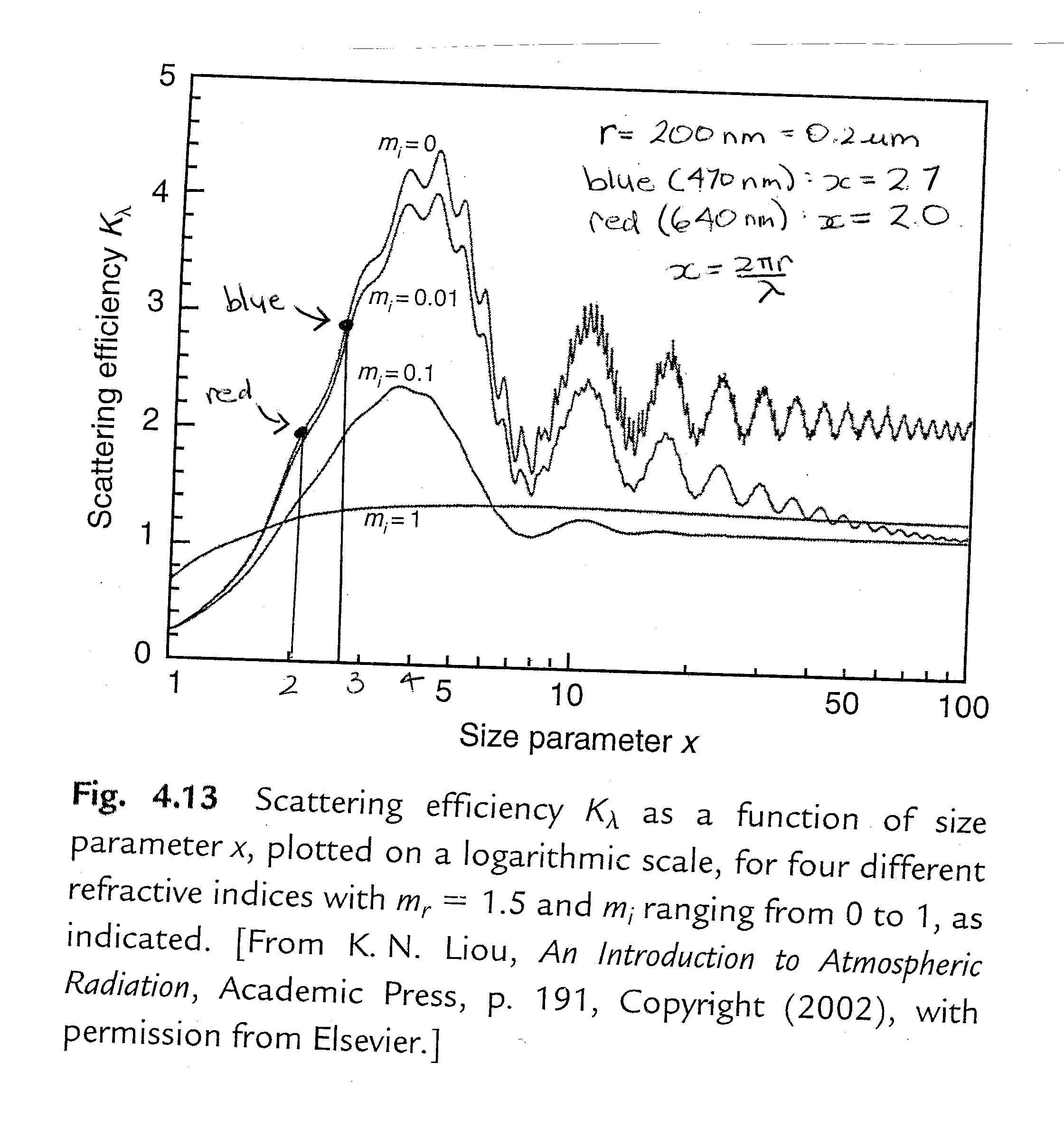# UV Absorption by Ozone

You should know how to use (4.17) to calculate the optical depth of an absorber or scatterer. For test questions, you will be able to assume that the mixing ratio r, or mass absorption coefficient are independent of height. Otherwise the problems are not easily solvable. You should be able to go between the optical depth, transmissivity, total mass/area of an absorber, average mass mixing ratio of the absorber. Given below is a typical problem of this type. The thickness of the ozone layer generally ranges from 220 DU in the tropics to a maximum of 450 DU in mid-latitudes.